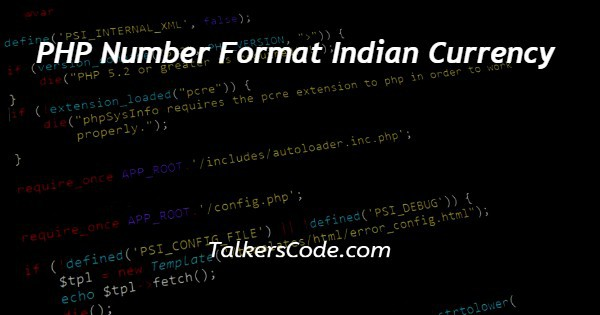# PHP Number Format Indian Currency

Last Updated : Jan 1, 2023In this tutorial we will show you the solution of PHP number format Indian currency, in php we have inbuilt method but that’s not work on windows so we manually calculates the Indian currency format.

As we know what is indian currency counting method from our childhood so we have implement that with the help of some of inbuilt php method.

## Step By Step Guide On PHP Number Format Indian Currency :-

For converting number to indian currency format we used substr(), strlen() and str_split() like those methods.

Those are used for to process the user inputs like substr() method for separate some part of integers from whole number, strlen() method used for collects overall digits present in our input number and str_split() function used for splitting number by some count.

Our program will works on whole digit number only can’t work on float digits. Result will display on plain webpage because we are not styled.

```<?php
\$amount = '1339000';
\$amount = moneyFormatIndia( \$amount );
echo \$amount;
function moneyFormatIndia(\$num) {
\$explrestunits = "" ;
if(strlen(\$num)>3) {
\$lastthree = substr(\$num, strlen(\$num)-3, strlen(\$num));
\$restunits = substr(\$num, 0, strlen(\$num)-3); // extracts the last three digits
\$restunits = (strlen(\$restunits)%2 == 1)?"0".\$restunits:\$restunits; // explodes the remaining digits in 2's formats, adds a zero in the beginning to maintain the 2's grouping.
\$expunit = str_split(\$restunits, 2);
for(\$i=0; \$i<sizeof(\$expunit); \$i++) {
// creates each of the 2's group and adds a comma to the end
if(\$i==0) {
\$explrestunits .= (int)\$expunit[\$i].","; // if is first value , convert into integer
} else {
\$explrestunits .= \$expunit[\$i].",";
}
}
\$thecash = \$explrestunits.\$lastthree;
} else {
\$thecash = \$num;
}
return \$thecash; // writes the final format where \$currency is the currency symbol.
}
?>```
1. A php script can be placed anywhere in the document. A php script starts with <?php and end with ?> tags.
2. The default file extension for php files is “.php” and php each statements end with ‘;’ semicolon. Between start and end tag we need to define our program in php.
3. We declared one variable name ‘\$amount’ with our input number and here when declare one variable we need to use ‘\$’ symbol before variable name.
4. In our program we take it ‘133000’ as input then we called function ‘moneyFormatIndia()’ with our input as parameter.
5. In function moneyFormatIndia() we defined our Indian rupee convertion let see each lines. ‘\$explrestunits’ this variable defined with empty string for later use.
6. Then we checked if() condition in our condition we checking whether our input length greater than 3 then we can do next steps.
7. If our number contain only 3 digits means it returns to function call and print on webpage because we don’t do more process for 3 digit number.
8. If our input is more than 3 digit we must do some process. First we need to collect last 3 digits from input by ‘substr()’ method and stored to variable ‘\$lastthree’.
9. Remaining part of numbers separated by same substr() function and stored to variable ‘\$restunits’ and with that we adding ‘0’ to front of remain part. The ‘\$restunits’ values grouped as two two of values then stored to ‘\$expunit’.
10. For looping all digits and append all values together we used for() loop it will process until it is size exit. If our digit is ‘0’ means we reach at most first digit of input so we need to change the value type to integer to avoid meaningless at front and add (,) after that digit.
11. If our digit is not ‘0’ then we need to append all digits together with delimiter (,) then the result stored to variable ‘\$explrestunits’. After end of for loop we need append those separated 3 digit at last position of result.
12. Then result will returns to function and printed on our webpage using ‘echo()’. It also a statement for print any values in php.

## Conclusion :-

In conclusion we are able to know how to push key with value to an array using php.

When work with php we need to create and process php files at server location and then we need to start the server before execute the program.

When executing this program on browser page, our input integer converted to our Indian currency format then printed the result.

I hope this tutorial on PHP number format Indian currency helps you and the steps and method mentioned above are easy to follow and implement.